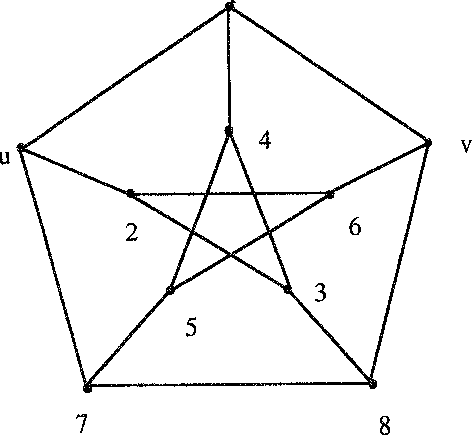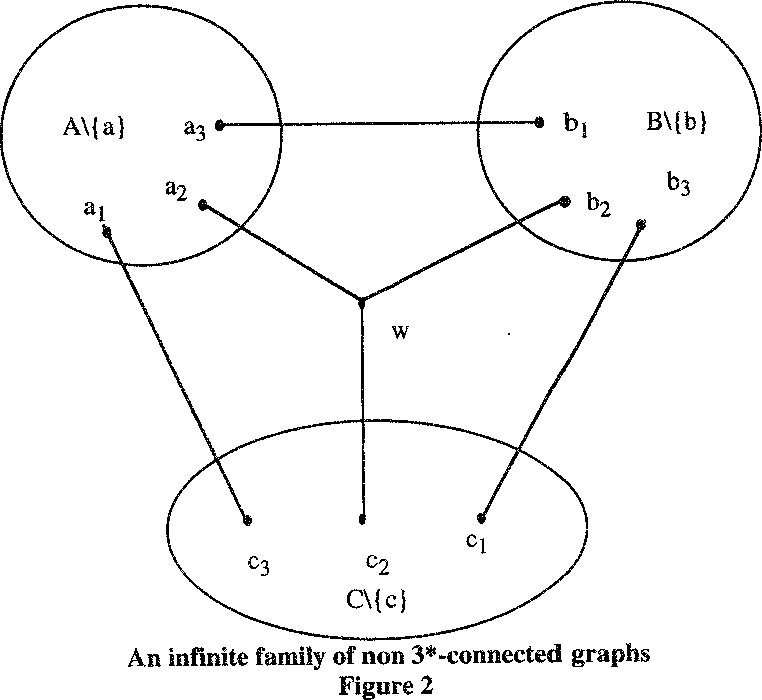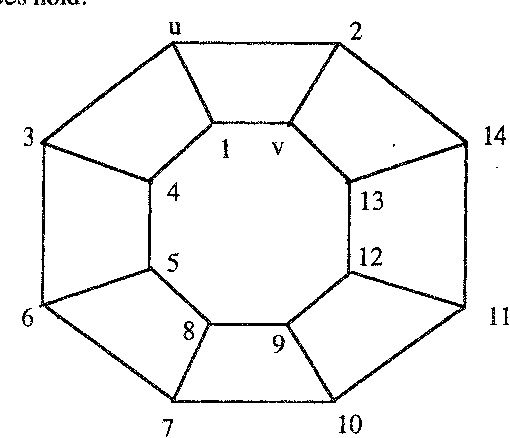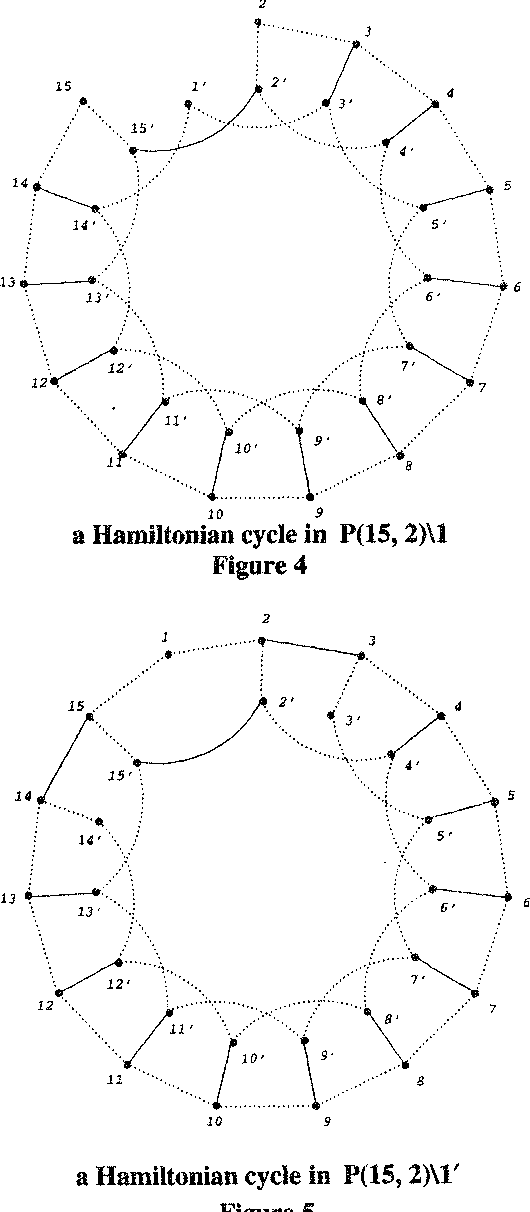You are currently offline. Some features of the site may not work correctly.

# Distance-regular graph

Known as: Intersection Array, Array, Distance-regular graphs
In mathematics, a distance-regular graph is a regular graph such that for any two vertices v and w, the number of vertices at distance j from v and… Expand
Wikipedia

## Papers overview

Semantic Scholar uses AI to extract papers important to this topic.
Highly Cited
2007
Highly Cited
2007
• 2007
• Corpus ID: 116439499

•••••Is this relevant?
1999
1999
• 1999
• Corpus ID: 5823672
We show that each distance-regular graph of valency four has known parameters.
Is this relevant?
1999
1999
We prove the following theorem. \emsp;Theorem.Let ?=(X, R)denote a distance-regular graph with classical parameters(d, b, ?, s… Expand
Is this relevant?
Highly Cited
1997
Highly Cited
1997
• J. Comb. Theory, Ser. B
• 1997
• Corpus ID: 6589087
The local adjacency polynomials can be thought of as a generalization, for all graphs, of (the sums of ) the distance polynomials… Expand
Is this relevant?
Highly Cited
1996
Highly Cited
1996
• J. Comb. Theory, Ser. B
• 1996
• Corpus ID: 18339226
The concept of local pseudo-distance-regularity, introduced in this paper, can be thought of as a natural generalization of… Expand
Is this relevant?
Highly Cited
1995
Highly Cited
1995
Given a nontrivial primitive idempotent E of a distance-regular graph ? with diameter d ? 3, we obtain an inequality involving… Expand
Is this relevant?
Highly Cited
1992
Highly Cited
1992
• J. Comb. Theory, Ser. B
• 1992
• Corpus ID: 17688335
Distance regular graphs fall into three families: primitive, antipodal, and bipartite. Each antipodal distance regular graph is a… Expand
Is this relevant?
1987
1987
• J. Comb. Theory, Ser. B
• 1987
• Corpus ID: 33293920
One problem with the theory of distance-regular graphs is that it does not apply directly to the graphs of generalised polygons… Expand
Is this relevant?
1986
1986
Let G be a bipartite distance-regular graph with bipartition V ( G ) = X ∪ Y . Let V ( G ′) = X and, for x and y in X , let x be… Expand
Is this relevant?
1983
1983
A diameter-bound theorem for a class of distance-regular graphs which includes all those with even girth is presented. A new… Expand
Is this relevant?